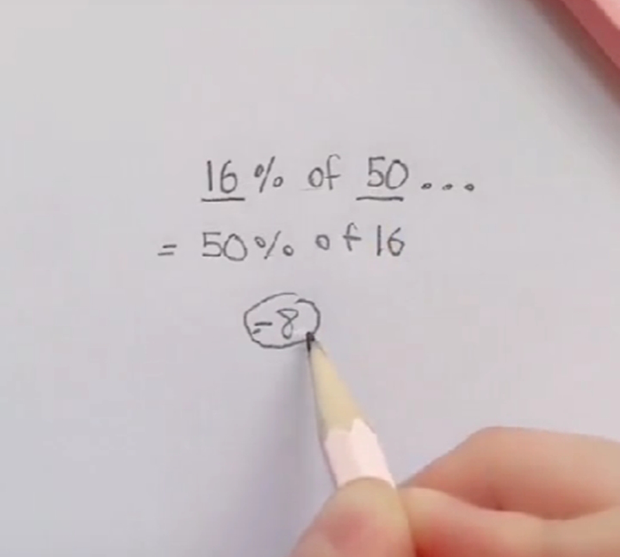# Quick calculation: "16% of 50 is?…" – The solution attracts 1.2 million followers, even elementary school children can understand it!

A complicated math, but just think a little differently, you can completely do it.

0
841

Have you ever found that calculations are extremely difficult, complicated, and difficult to solve quickly, especially problems about calculating percentage values. If the answer is yes, then don't worry, the calculation trick below will help you solve the problem in an extremely interesting and accurate way.

Specifically, the question asked to calculate 16% of 50 = how much? Instead of calculating the normal way, or click the calculator. A TikToker of 1.2 million followers who regularly posts videos about tips and tricks to make Math easier, has shown another way to do the math.

Instead of the usual calculation, the account that posted the video above has put this calculation into the case that it is equivalent to 50% of 16, which is equal to 8.A very unique way to calculate percentages

If you do a mental calculation of 16% of 50, it is true that this calculation is very difficult, and difficult to calculate quickly. But when switching to 50% of 16, anyone can easily calculate the result as 8.

The simple explanation is as follows:

16% of 50 = 16/100 * 50 = 16 * 50/100 => The result to be calculated is 50% of 16

Calculations that don't involve the number 50 can be difficult to do mentally, but the rule is still valid, giving accurate results. For example, a simple calculation:

20% of 50 = 10 and 50% of 20 = 10

Very quick and doesn't even need to calculate drafts right? Now you can quickly do percentage calculations. Let's try to quiz your friends around percentage calculations and see who can answer the questions faster!

Source: Popsugar

https://kenh14.vn/tinh-nhanh-16-cua-50-la-cach-giai-thu-hut-12-trieu-nguoi-theo-doi-den-tre-tieu-hoc-nhin-qua- supply-understanding-duoc-2022030914293018.chn